Assuming the sun to be a spherical body of radius R at a temperature of TK, evaluate the total radiant power, incident on earth, at a distance r from the sun.

Note: r0 is the radius of the earth and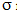is Stefan's constant.

Anonymous User Physics Heat Transfer 04 Apr, 2020 72 views

The figure shows a system of two concentric spheres of radii r1 and r2 and kept at temperatures T1 and T2, respectively. The radial rate of flow of heat in a substance between the two concentric spheres is proportional to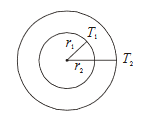Anonymous User Physics Heat Transfer 04 Apr, 2020 66 views

The temperature of the two outer surfaces of a composite slab, consisting of two materials having coefficients of thermal conductivity K and 2K and thickness x and 4x, respectively are T and T1  (T > T1). The rate of heat transfer through the slab, in a steady state is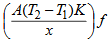, with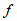equal to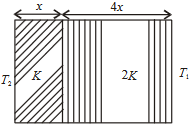Anonymous User Physics Heat Transfer 04 Apr, 2020 61 views

If the temperature of the sun were to increase from T to 2T and its radius from R to 2R, then the ratio of the radiant energy received on earth to what it was previously will be

Anonymous User Physics Heat Transfer 04 Apr, 2020 67 views

According to Newton's law of cooling, the rate of cooling of a body is proportional to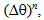where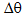is the difference of the temperature of the body and the surroundings, and n is equal to

Anonymous User Physics Heat Transfer 04 Apr, 2020 83 views

Two spheres of the same material have radii 1 m and 4 m and temperatures 4000 K and 2000 K respectively. What is the ratio of the energy radiated per second by the first sphere to that by the second?

Anonymous User Physics Heat Transfer 04 Apr, 2020 58 views

One end of a thermally insulated rod is kept at a temperature T1 and the other at T2. The rod is composed of two sections of lengths l1 and l2 and thermal conductivities K1 and K2 respectively. What would be the temperature at the interface of the two sections?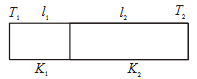-0

Anonymous User Physics Heat Transfer 04 Apr, 2020 62 views

A Carnot engine, having an efficiency of n = 1/10 as a heat engine, is used as a refrigerator. If the work done on the system is 10 J, what will be the amount of energy absorbed from the reservoir at a lower temperature?

Anonymous User Physics Thermodynamics 04 Apr, 2020 56 views

When a system is taken from state i to state f along the path iaf, it is found that Q = 50 cal and W = 20 cal. Along the path ibf Q = 36 cal. W along the path ibf is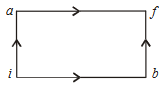Anonymous User Physics Thermodynamics 04 Apr, 2020 61 views

Two rigid boxes containing different ideal gases are placed on a table. Box A contains one mole of nitrogen at temperature T0, while Box B contains one mole of helium at temperature (7/3) T0. The boxes are then put into thermal contact with each other and heat flows between them until the gases reach a common final temperature. (Ignore the heat capacity of boxes). Then, what will be the final temperature of the gases, Tf, in terms of T0?

Anonymous User Physics Thermodynamics 04 Apr, 2020 61 views

The work of 146 kJ is performed in order to compress one kilo mole of gas adiabatically and in this process the temperature of the gas increases by 7ºC. The gas is (R = 8.3 J mol-1 K-1)

Anonymous User Physics Thermodynamics 04 Apr, 2020 62 views

A system goes from A to B via two processes I and II as shown in the below figure. If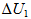and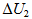are the changes in internal energies in the processes I and II respectively, then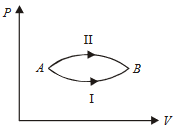Anonymous User Physics Thermodynamics 04 Apr, 2020 56 views

The temperature­ entropy diagram of a reversible engine cycle is given in the figure. Its efficiency is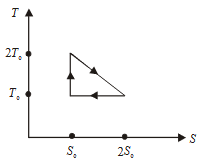Anonymous User Physics Thermodynamics 04 Apr, 2020 59 views

Two thermally insulated vessels 1 and 2 are filled with air at temperatures (T1, T2 ), volume (V1, V2 ), and pressure (P1, P2 ), respectively. If the valve joining the two vessels is opened, the temperature inside the vessel at equilibrium will be

Anonymous User Physics Thermodynamics 04 Apr, 2020 77 views

One mole of ideal monoatomic gas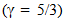is mixed with one mole of diatomic gas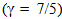. What is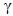for the mixture?

Note:denotes the ratio of specific heat at constant pressure, to that at constant volume.

Anonymous User Physics Thermodynamics 04 Apr, 2020 57 views

During an adiabatic process, the pressure of a gas is found to be proportional to the cube of its absolute temperature. The ratio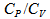for the gas is

Anonymous User Physics Thermodynamics 04 Apr, 2020 54 views

1 mole of a gas with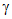= 7/5 is mixed with 1 mole of a gas with= 5/3, then what is the value offor the resulting mixture?

Anonymous User Physics Thermodynamics 04 Apr, 2020 59 views